# JavaScript Arrow Function

## Arrow Functions in JavaScript with Examples

In this article, we will discuss Arrow Functions in JavaScript with Examples. Please read our previous article where we discussed JavaScript Immediately Invoked Function Expressions (IIFE). First, we will discuss what is an arrow function, its syntax, and its structure. Then we will clarify one myth that the arrow functions will help you to make your code more readable, which is not true. The important use of the arrow function is that it helps us to implement automatic current context, which we will also discuss in this article. And last, we will discuss the Bind method which is an alternative to the arrow functions.

##### What are JavaScript Arrow Functions?

The arrow function was introduced in ECMAScript 6(ES6) that provides a concise and shorthand for declaring an anonymous function. The Arrow Functions in JavaScript helps us to create anonymous functions or methods i.e. functions without name. As they do not have any names, the arrow makes the syntax shorter.

1. Arrow functions are a concise way of writing anonymous, lexically scoped functions in ECMAScript 2015 (ES6).
2. The Arrow functions can contain other arrow functions or also regular functions.
3. Arrow function accomplishes the same result as regular function with fewer lines of code.
4. The arrow function automatically binds this object to the surrounding code’s context.
5. The value of this keyword inside the arrow function is not dependent on how they are called or how they are defined. It depends only on its enclosing context.
6. If the arrow function is used as an inner function this refers to the parent scope in which it is defined.
##### How to convert Normal function to Arrow function in JavaScript?

An Arrow function is very similar to a regular function in behavior but is quite different syntactically. Let us understand this with an example. Please have a look at the below example. Here, we have one Add method which takes two parameters and then adds the two parameters values, and then returns the results from where it is being called. And then we call the Add method and log the result in the console.

```<html>
<title>JavaScript Arrow Functions Example</title>
<body>
<script>
{
return num1 + num2;
}
console.log("Sum of 10 and 20 is : "+ Add(10, 20))
</script>
</body>
</html>
```

Output: Sum of 10 and 20 is : 30

Let us see how to convert the above Add function to an arrow function in JavaScript. Please have a look at the below image. As you can see in the below image, you need to write a pair of round brackets () where you need to place the input parameters if any and then you need to write the arrow symbol (=>) followed by a pair of curly braces. Within the curly braces, you need to write your logic including the return statement if any.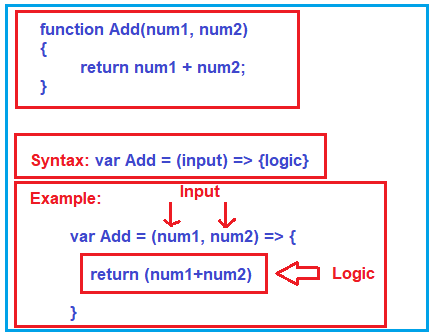###### The complete example is given below.
```<html>
<title>JavaScript Arrow Functions Example</title>
<body>
<script>
var Add = (num1, num2) => {
return (num1+num2)
}
console.log("Sum of 10 and 20 is : "+ Add(10, 20))
</script>
</body>
</html>
```

Output: It will also print the same output as the previous example. Run the application and open the browser console window by pressing the F12 key and you should see the following output as expected.

Sum of 10 and 20 is : 30

##### Myth: The arrow function makes the code readable and simple.

Now the question what are the advantages of using the arrow function. Is really the arrow makes the code smaller, more readable, and simple? If you ask this question to me, then personally I don’t feel so that the arrow function makes the code simple and more readable. You just have a look at the following image where I am showing the same Add function using Arrow and without using the Arrow function.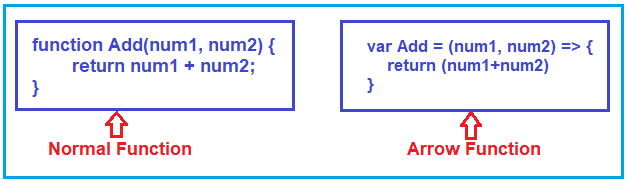##### When we should use Arrow Functions in JavaScript?

Arrow functions in JavaScript should be used when you want to run your code in Caller’s Context. That is when you want to ensure your code runs under the caller’s context, then you should use Arrow Functions in JavaScript.

##### What is a Context in JavaScript?

It is a little complex to understand the concept context in JavaScript. Let us try to understand what is context in JavaScript. Let us understand this with an example. Suppose you created the following context variable in your code globally.

<script>
this.context = “Global”;
</script>

When the application runs i.e. the window runs of your browser, the above object (this.context) created globally. For better understanding, please have a look at the following image.Now, you can access the global context object anywhere within the page. You can access directly or even you can access within any function as shown in the below example.

```<html>
<title>JavaScript Arrow Functions Example</title>
<body>
<script>
this.context = "Global";
function fun1()
{
}
console.log(this.context);
fun1();
</script>
</body>
</html>
```

So, everything is good till here. But if you create a new instance using the new keyword, then for that new instance automatically a new this will be created as shown in the below image.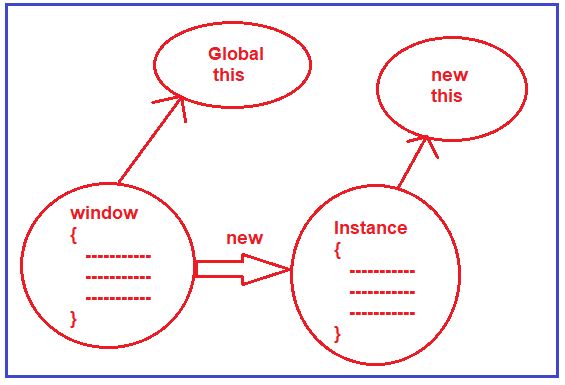Little confusing right. So, let us see an example to understand this concept. Please have a look at the below example. Here, first, we are calling the fun1 function and it will display the output i.e. it will print this.context value as expected. Then we created a new instance of this fun1 function and this time it will print undefined. This because the new keyword creates a new instance and for that new instance a new this will be created.

```<html>
<title>JavaScript Arrow Functions Example</title>
<body>
<script>
this.context = "Global";

function fun1()
{
console.log(this.context);
}
fun1(); //Showing Global
var instance = new fun1(); //Showing undefined

</script>
</body>
</html>
```
###### Output: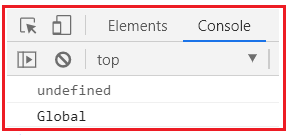Now if we create this.context within the function and set its value to local, then it will print the value local as shown in the below example.

```<html>
<title>JavaScript Arrow Functions Example</title>
<body>
<script>
this.context = "Global";

function fun1()
{
this.context = "Local";
console.log(this.context);
}
console.log(this.context); //Showing Global
var instance = new fun1(); //Showing Local

</script>
</body>
</html>
```

Note: The point that you need to remember is that both the “this” are different now. We have a global “this” context created by the browser and when we use the new keyword, we have a separate new “this” created.

Let us make our code a little complicated. Please have a look at the following example. Here, we have created another function called Fun2, and this function is called from the body of the Fun2 function. This function is also accessing this.context object. Then we created an instance of the Fun1 function.

```<html>
<title>JavaScript Arrow Functions Example</title>
<body>
<script>
this.context = "Global";

function fun1()
{
this.context = "Local";
console.log("Within Fun1 Function : "+ this.context);
fun2();
}

function fun2()
{
console.log("Within Fun2 Function : "+ this.context);
}
var instance = new fun1();

</script>
</body>
</html>
```

Here, our expectation is it will print the value local in both the places (with fun1 and fun2 functions). Why this expectation is because the caller of the Fun2 function is the fun1 function and we are creating a new instance of the fun1 function. So, any method which is invoked through the new instance should have the same this.context.

Now run the application. Open the browser console window and see the output. The output is not as per our expectations. Within the fun1 function, it is printing the value local which is fine. But what happens inside the Fun2 function i.e. why it is printing the value Global. This is because, when the fun1 function calls the fun2 function, the fun2 function just lost the context and starts referring to the global context. This is confusing.

##### How to retain the context object of the caller?

We would like to retain the context object of the caller and this is possible by using the Arrow function in JavaScript and I think this is the only place where you need to use the arrow function in your project. The arrow function runs inside the context of the caller. For better please have a look at the below code.

```<html>
<title>JavaScript Arrow Functions Example</title>
<body>
<script>
this.context = "Global";

function fun1()
{
this.context = "Local";
console.log("Within Fun1 Function : "+ this.context);
var fun2= () => {
console.log("Within Fun2 Function : "+ this.context);
};
fun2();
}
var instance = new fun1();
</script>
</body>
</html>
```

Now save the changes and run the application and should the get the output as expected in the browser console window as shown in the below image.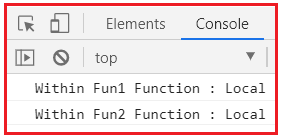So, when you call an arrow function, he does not have any context, instead, it will run inside the caller’s context.

##### Arrow Functions Alternative: Bind Function in JavaScript

So. if you have requirements where you have explicitly binding the context i.e. running the code inside the caller’s context, then having an arrow function is good. But before the arrow function, developers use the bind function. They call the bind method and to the method, they just pass the context object (this) as shown in the below example. Here, in the below example, we are appending the bind function to the Fun2 function, and to the bind method, we are passing the context object as a parameter.

```<html>
<title>JavaScript Arrow Functions Example</title>
<body>
<script>
this.context = "Global";

function fun1()
{
this.context = "Local";
console.log("Within Fun1 Function : "+ this.context);
var x = fun2.bind(this);
x();
}

function fun2()
{
console.log("Within Fun2 Function : "+ this.context);
}

var instance = new fun1();

</script>
</body>
</html>
```

Now, if you will run the application, then also you will get the same output as the previous example which we have done using the arrow function.

##### Points to Remember:
1. Do Not use the Arrow Functions in JavaScript to make your code simpler and readable. On the contrary, it can make your code more complicated.
2. Use Arrow functions when you want to run your code in the current context.
3. Do not use arrow functions until you need it really. Normal functions with Bind look more readable and simpler.
##### Arrow function Basic syntax rule for different scenario

Basic syntax rules for an arrow function are as follows:

Arguments should be passed in the small parentheses (). For only one argument, parentheses are optional. For zero argument or without arguments, there must be an empty parenthesis. If there is a single expression or statement in the function body

1. Using parenthesis is optional
2. Using the return statement is optional

There is a special syntax to return an object.  Either an object should be enclosed inside a small bracket ({}) or should be returned using the return statement. Let’s explore each one of the above-mentioned arrow function’s basic syntax rules for different conditions in detail with example.

##### Arrow function without parameter /zero Argument in JavaScript

If an Arrow function takes no parameter or Arrow function with no parameters, parentheses () will be empty but they should be present.

###### Example:
```<html>
<title>JavaScript Arrow function without any parameter example</title>
<body>
<script>
//Normal function
function intro() {
return "Welcome to Normal function without any parameter";
}
console.log(intro());

//Arrow function
let arrowStarup = () => "Welcome to Arrow function without any parameter";
console.log(arrowStarup());
</script>
</body>
</html>
```
###### Output: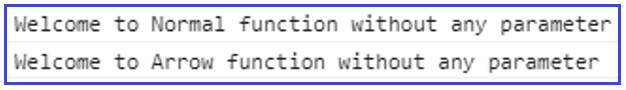In the above example, we have removed the ‘function’ and replace it with a variable assignment, in the above example arrowStartup. The arrow functions are easier to read and have cleaner syntax.

##### JavaScript Arrow function with one parameter

If an Arrow function having one parameter/argument, the parentheses () around that parameter are optional and aren’t required. If we want, we can remove the parentheses.

##### Syntax:

One param. With simple expression, the return is not needed. param => expression

###### Example:
```<html>
<title>JavaScript Arrow function with one parameter example</title>
<body>
<script>
//Normal function
function isNegative(number) {
return number <= 0
}
console.log("Normal function: ", isNegative(3));

//Arrow function having one parameter without parentheses
let arrowIsNegative = number => number <= 0;

//Arrow function having one parameter with parentheses
let arrowIsNegativeWithParn = (num) => num <= 0;

console.log("Arrow function :", arrowIsNegative(-1));
console.log("Arrow function with Parentheses :", arrowIsNegativeWithParn(5));
</script>
</body>
</html>
```
###### Output: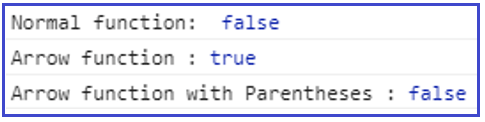In the above example snippet, since there is 1 parameter so we can remove the parentheses around the parameter number. Again, the functions are exactly the same.

##### JavaScript Arrow function with multiple parameters

If the Arrow function has multiple arguments or no argument, we must put parentheses () around the arguments.

###### Syntax:

Multiple params require parentheses. With simple expression return keyword is not needed: (param1, param2, paramN) => expression

###### Example: JavaScript Arrow function with multiple parameters Examples
```<html>
<title>JavaScript Arrow function with Multiple and No parameters example</title>
<body>
<script>
//Normal function
function total(a, b) {
return a + b;
}
console.log("Normal function multiple param: ", total(5, 7));

//Arrow function having multiple parameters
let arrowTotal = (a, b) => a + b;
console.log("Arrow function multiple param :", arrowTotal(11, 14));

//Normal Function (no arguments)
let a = 4; let b = 2;
function sum() {
return a + b + 100;
}
console.log("Normal function with no param:", sum());

//Arrow Function (no arguments)
let x = 4; let y = 2;
let arrowSum = () => x + y + 100;
console.log("Arrow function with no param:", arrowSum());
</script>
</body>
</html>
```
###### Output: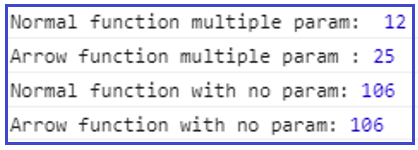Everything after the arrow => is assumed to be returned. a+b with multiple parameters will be returned because there aren’t any brackets { } or return statements and the same happen for x+y with no parameter also. The above fours are exactly the same functions, just the second and fourth ones is using arrow function syntax.

##### Arrow function with single Statement

Let’s see the example of the Arrow function body having a single statement

```//Without Arrow Function
hello = function () {
return "JavaScript Tutorial";
}

//With Arrow Function
hello = () => {
return "JavaScript Tutorial";
}
```

It gets shorter!

If the Arrow function body has only one statement, and the statement returns a value, we can remove the brackets { } and the return keyword and write all on a single line hello = () => “JavaScript Tutorial”;

##### JavaScript Arrow function with multiple statements

If the Arrow function body needs multiple lines of statements or expression, we must enclose them in curly braces { } also use a return keyword within them to return a value. If we use curly braces, then we need an explicit “return” keyword else it will not return a value rather show undefined

##### Syntax:

Multiple params require parentheses. Multiline statements require body brackets and return:

```(param1, paramN) => {
let a = 1;
return a + param1 + paramN;
}
```
###### Example: JavaScript Arrow function with Multiple statements/expression example
```<html>
<title>JavaScript Arrow function with Multiple statements/expression example</title>
<body>
<script>
//Normal function
function multiStatmnt(a, b) {
let chunk = 100;
return a + b + chunk;
}
console.log("Normal function multiple statements: ", multiStatmnt(5, 7));

//Arrow function having multiple statements/expression
let arrowMultiStatmnt = (a, b) => {
let chunk = 100;
return a + b + chunk;
};
console.log("Arrow function multiple statements :", arrowMultiStatmnt(11, 14));

//Arrow function having multiple statements without return keyword
let arrowMultiStatmntNoRetrn = (a, b) => {
let chunk = 100;
a + b + chunk;
};
console.log("Arrow function multiple statements without Return keyword:", arrowMultiStatmntNoRetrn(11, 14));

</script>
</body>
</html>
```
###### Output: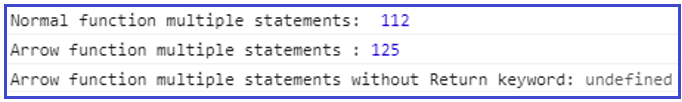Arrow functions are a one-liner function. They come in with two suggestion

Without curly braces: (…args) => function expression – the right-hand side is an expression. The function executes it and returns the desired result

With curly braces: (…args) => {function body } – the brackets allow us to write multiple statements inside the function but that needs an explicit return keyword to return something.

##### Arrow function as Anonymous function in JavaScript

An Arrow function can be used with the Anonymous function to attached addEventListener to button control.

###### Example: JavaScript Arrow function with Anonymous function example
```<html>
<title>JavaScript Arrow function with Anonymous function example</title>
<body>
<button id="myNormalBtn">Normal Function-Click Me</button>
<button id="myArrowBtn">Arrow Function-Click Me</button>

<script>
//Normal function
console.log('Normal function was clicked');
})

//Arrow function with Anonymous function
console.log('Arrow function was clicked');
})
</script>
</body>
</html>
```
###### Output: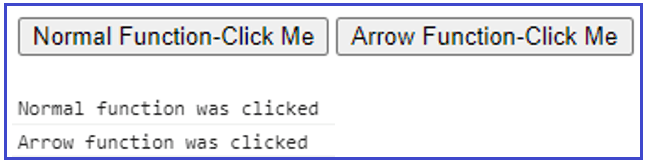In the above code, the Arrow function attached as an Anonymous function is fired when we clicked on the button which is the same as when clicking on the Normal function button.

##### Arrow function with the map method
###### Example:
```<html>
<title>JavaScript Arrow function with map() method example</title>
<body>
<script>
//Normal function
let arr = [1, 2, 3]
let squares = arr.map(function (x) {
return x * x;
});
console.log('Normal function map(): ', squares);

//Arrow function with map() method
var array = [1, 2, 3]
var arrowSquares = array.map(x => x * x);
console.log('Arrow function map(): ', arrowSquares)
</script>
</body>
</html>
```
###### Output: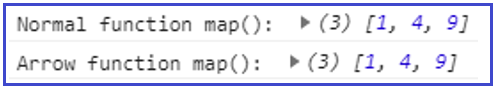##### Arrow function with Duplicate Named Parameter

While passing the Duplicate Named Parameter to the method is allowed in the Regular function. When in “use strict” mode, this will throw Error: Syntax Error: Duplicate parameter name not allowed in this context.

Whereas passing the Duplicate Named Parameter to the method is not allowed in the Arrow function. It will throw Error regardless of strict or non-strict mode.

##### Example: Normal function with Duplicate Named Parameter
```<html>
<title>JavaScript Normal function with Duplicate Named Parameter example</title>
<body>
<script>
//Normal function
function multiply(a, a) {
return a * a;
}
console.log("Normal function Duplicate Named Parameter : ", multiply(5, 7));
</script>
</body>
</html>
```

Output: Normal function Duplicate Named Parameter : 49

##### Example: Arrow function with Duplicate Named Parameter
```<html>
<title>JavaScript Arrow function with Duplicate Named Parameter example</title>
<body>
<script>
//Arrow function having Duplicate Named Parameter
let arrowMultiply = (a, a) => a * a;
console.log("Arrow function Duplicate Named Parameter :", arrowMultiply(11, 14));
</script>
</body>
</html>
```
###### Output: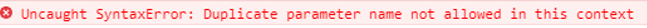##### JavaScript Array Function Returning Object Literals

We need to keep in mind that while returning object literals with Arrow Function using the concise body syntax i.e.: params => { object: literal } will not work as expected. To understand this, see below example

```<html>
<title>JavaScript Arrow function Returning Object literals example1</title>
<body>
<script>
var arrowFunc = () => { price: 10 };
// Calling func() returns undefined!
console.log("Arrow function(arrowFunc) Returning Object literal :", arrowFunc());

var arrowFunc1 = () => { price: function() { } };
// SyntaxError: function statement requires a name
console.log("Arrow function(arrowFunc1) Returning Object literal :", arrowFunc1());

</script>
</body>
</html>
```
###### Output: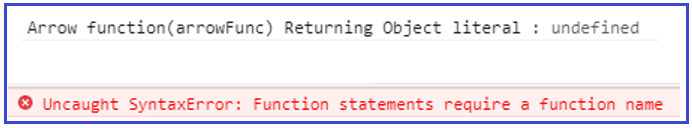This is because the code placed inside the curly bracket ({}) is parsed as a sequence of an element that is treated as a label, not as a key in an object literal. We must wrap the object literal in parentheses like- ({ object: literal })

##### Example: JavaScript Arrow function Returning Object literals example
```<html>
<title>JavaScript Arrow function Returning Object literals example2</title>
<body>
<script>
//Normal function
var objectReturn = function objectReturn(x) {
return { price: 100 + x };
}
console.log("Normal function Returning Object literal : ", objectReturn(5));

//Arrow function Returning Object literals
let arrowObjectReturn = x => ({ price: 100 + x })
console.log("Arrow function Returning Object literal :", arrowObjectReturn(5));

</script>
</body>
</html>
```
###### Output: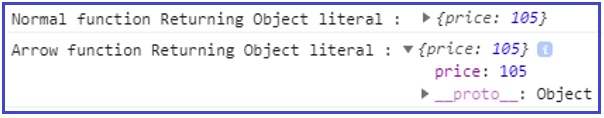##### JavaScript Arrow Function Returning

While returning the function from arrow functions it displays undefined in place of function. This is due to the empty arrow function as the Empty arrow function returns undefined. But why this is returning will see. First, go through the example first.

###### Example:
```<html>
<title>JavaScript empty Arrow function and Returning value example</title>
<body>
<script>
//Arrow function returning value
let arrowRetnVal = () => 10;
console.log("Arrow function Returning value : ", arrowRetnVal());

//An empty arrow function returns undefined
let arrowRetnFunc = () => { };
console.log("Arrow function Returning function : ", arrowRetnFunc());
</script>
</body>
</html>
```
###### Output: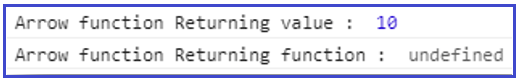We might expect {} instead of undefined. This is because the curly braces are part of the syntax of the arrow functions, so arrowRetnFunc will return undefined. It is possible to return the {} object directly from an arrow function, by enclosing the return value with brackets.

##### Example:
```<html>
<title>JavaScript Arrow function, Function Returning empty object two ways example</title>
<body>
<script>
//Arrow function
let arrowRetnFunc1 = () => ({});
console.log("Arrow function Returning function-1 : ", arrowRetnFunc1());

let arrowRetnFunc2 = () => { return {} };
console.log("Arrow function Returning function-2 : ", arrowRetnFunc2());
</script>
</body>
</html>
```
###### Output: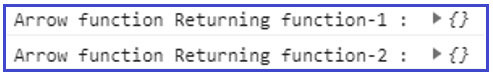If we need to return an empty object from an arrow function, we can return it in one of two ways mentioned in the code snippet.

##### JavaScript Arrow Function Implicit Return

Arrow functions can implicitly return values by simply omitting the curly braces that traditionally wrap a function’s body if their body only contains a single expression. That means an Implicit return keyword is not needed with one or multiple params with a single expression or body statement.

##### Example: Arrow function Implicit Return
```<html>
<title>JavaScript Arrow function Implicit Return example</title>
<body>
<script>
//Arrow function simple Implicit Return
let arrowImplicitReturn = x => x * x + 10;
console.log("Arrow function Implicit Return : ", arrowImplicitReturn(5));
</script>
</body>
</html>
```

Output: Arrow function Implicit Return : 35

When using implicit returns, object literals must be wrapped in parenthesis so that the curly braces are not mistaken for the opening of the function’s body.

##### Example: Arrow function Implicit Return with Object literals
```<html>
<title>JavaScript Arrow function Implicit Return with Object literals example</title>
<body>
<script>
//Arrow function Implicit Return with object literals without parentheses
let arrowImplctRetrn_objLitrls_1 = () => { price: 10 } //returns undefined
console.log("Arrow function Implicit Return without parentheses : ", arrowImplctRetrn_objLitrls_1());

//Arrow function Implicit Return with object literals with parentheses
let arrowImplctRetrn_objLitrls_2 = () => ({ price: 10 })
console.log("Arrow function Implicit Return with parentheses : ", arrowImplctRetrn_objLitrls_2());

</script>
</body>
</html>
```
###### Output: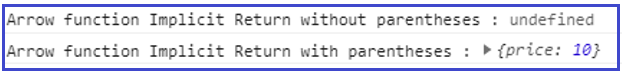##### Array Function Explicit Return in JavaScript

Arrow functions can behave very similar to classic Regular/Named functions in that you may explicitly return a value from them using the return keyword; simply wrap your function’s body in curly braces, and return a value:

###### Example:
```<html>
<title>JavaScript Arrow function Explicit Return example</title>
<body>
<script>
//Arrow function Explicit Return
let arrowExplicitReturn = x => {
return x * x + 10;
}
console.log("Arrow function Explicit Return : ", arrowExplicitReturn(5));

</script>
</body>
</html>
```

Output: Arrow function Explicit Return :35

##### Arguments Object binding

Regular functions have arguments binding. Arrow functions do not have arguments binding. An arrow function is a lightweight version of Regular functions with a focus on being short and lexical this. Simultaneously, arrow functions do not provide a binding for their own arguments object.

As a valid alternative use the rest parameters to achieve the same result. Arrow functions do not have their own arguments object; therefore, arguments would simply refer to a variable pass in the current scope. Because of this, arrow functions are also not aware of their caller/callee.

As the lack of an argument object can be a limitation in some edge cases, rest parameters are generally a suitable alternative.

##### Example: Arrow function Arguments binding
```<html>
<title>JavaScript Arrow function Arguments binding example</title>
<body>
<script>
//Normal function
let argsBinding = function () {
return arguments;
}
console.log("Normal function arguments binding : ", argsBinding("5", 6, 7));

//Arrow function arguments Binding
let arrowArgsBinding = () => arguments
console.log("Arrow function arguments binding: ", arrowArgsBinding("5", 6, 7));

</script>
</body>
</html>
```

Output: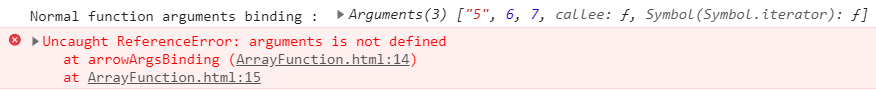In most of cases, using rest parameters is the best alternative to using an argument object binding.

##### Example: Arrow function Arguments binding with rest parameters
```<html>
<title>JavaScript Arrow function Arguments binding using rest Parameters example</title>
<body>
<script>
//Arrow function arguments Binding using rest parameters
let arrowArgsBidng_RestPram = (...args) => args
console.log("Arrow function arguments Binding using rest parameters : ", arrowArgsBidng_RestPram("5", 6, 7));

</script>
</body>
</html>
```
###### Output: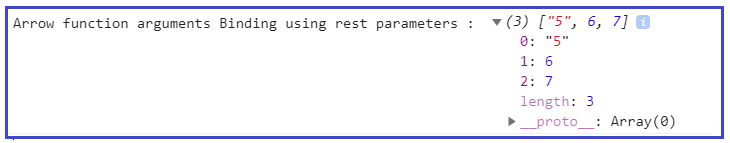##### Arrow function as a Constructor/ new Keyword

Regular functions can be used as constructor functions while Arrow functions cannot be used as constructor functions.

##### Why?

Regular functions created using function declarations or expressions are constructible and callable. Since regular functions are constructible, they can be called using a new keyword. Since arrow functions are not constructible, they can never be called using the new keyword. Arrow functions cannot be used as constructors as they will throw a TypeError when used with a new keyword.

##### Example: Arrow function with new keyword without parameter/ parameterless constructor
```<html>
<title>JavaScript Arrow function with new keyword without parameter example</title>
<body>
<script>
//Normal function
const normalFunc = function () {
return "normalFunc constructor";
}
const a = new normalFunc();
console.log("Normal function with new keyword: ", a);

//Arrow function with new keyword without parameter
const arrowfunc = () => {
return "arrowfunc constructor";
}
const b = new arrowfunc(); // -> Uncaught TypeError: arrowfunc is not a constructor...
console.log("Arrow function with new keyword: ", b);

</script>
</body>
</html>
```
###### Output: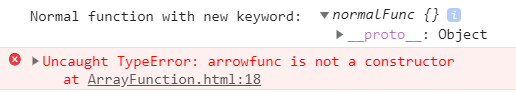##### Example: Arrow function with new keyword and parameter / parameterize constructor
```<html>
<title>JavaScript Arrow function with new keyword and parameter example</title>
<body>
<script>
//Normal function
function User(name, age, sex) {
this.name = name;
this.age = age;
this.sex = sex;
}
const Shagufta = new User("Shagufta", 23, "Female");
console.log("Normal function using new keyword with parameters: ", Shagufta);

//Arrow function with new keyword and parameter
const Person = (name, age, sex) => {
this.name = name;
this.age = age;
this.sex = sex;
}
const John = new Person("John", 23, "Male"); // -> Uncaught TypeError: John is not a constructor...
console.log("Arrow function using new keyword with parameters: ", John);

</script>
</body>
</html>
```
###### Output: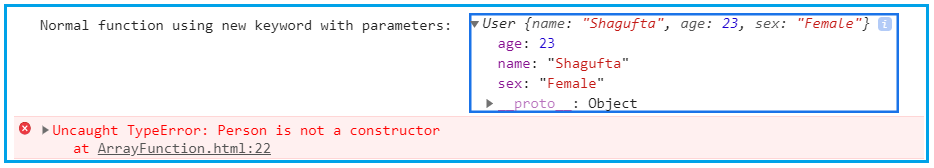From the above code, we can say that Arrow functions are callable but not constructible. Regular functions are callable and constructible.

##### JavaScript Arrow function with this keyword

What makes arrow function really impressive is the fact that they redefine ‘this’ keyword inside of them as opposed to normal function

With regular function, the value of ‘this’ is set based on how the function is called. With the arrow function, the value of ‘this’ is based on the function’s surrounding context. This means that the value of ‘this’ inside an arrow function is the same as the value of this outside the function.

Unlike a regular function, an arrow function does not bind this object. Instead, this is bound lexically i.e.: this keeps its meaning from its original context.

##### What do we mean by that?

The value of this keyword inside the arrow function is not dependent on how they are called or how they are defined. It depends only on its enclosing context. As we know that arrow functions are lexically scope.

##### What is lexical Scope?

Lexical Scope also called static scope. Every inner level can have access to its outer level.

###### Let us understand this with some examples:
```<html>
<title>JavaScript Arrow function Lexical Scope example</title>
<body>
<script>
var scope = "I am global";
function whatIsFuncScope() {
var scope = "I am just a local";
function func() { return scope; }
return func;
}

console.log("whatIsFuncScope-lexical Scope : ", whatIsFuncScope()());
</script>
</body>
</html>
```

Output: whatIsFuncScope-lexical Scope : I am just a local

The above code will return “I am just a local”. It will not return “I am a global”. Because the function func() counts where it was originally defined which is under the scope of function whatIsFuncScope.

It will not worry from whatever it is being called (the global scope/from within another function even), that’s why global scope value “I am global” will not be printed.

This is called lexical scoping where “functions are executed using the scope chain that was in effect when they were defined” – according to JavaScript Definition Guide. Lexical scope is a very powerful concept.

In the arrow function, this keyword refers to the object which made up the function. But in the Normal function, this keyword refers to the element which calls the function via event. Since all global variables and functions are inside the window. this keyword inside the global function will refer to the window object.

The ES6 arrow function cannot be bound to this keyword, so it will lexically go up in function scope, and use the value of this object in the scope in which it was defined. The Regular function has its own binding. The source of this is an important distinguishing aspect of arrow functions:

• Regular functions have a dynamic this; their value is decided by how they are called
• Arrow functions have a lexical this; its value is decided by the surrounding scope.

The following variables are all lexical inside arrow functions:

• arguments
• super
• this
• new.target

With a regular function, this represents the object that calls the function.

##### Example:
```<html>
<title>JavaScript Regular function this keyword example</title>
<body>
<p id="demo"></p>
<button id="btn">Click Me</button>

<script>
//Normal function
hello = function () {
document.getElementById("demo").innerHTML += this;
}

//The Window object calls the function

//A button object calls the function
</script>
</body>
</html>
```
###### Output: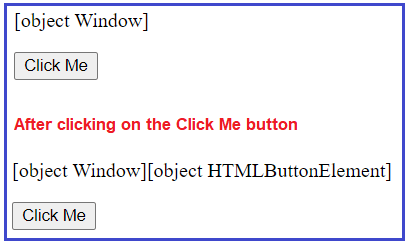With an arrow function, this represents the owner of the function

##### Example:
```<html>
<title>JavaScript Arrow function this keyword example1</title>
<body>
<p id="demo"></p>
<button id="btn">Click Me</button>

<script>
//Arrow function
hello = () => {
document.getElementById("demo").innerHTML += this;
}

//The Window object calls the function

//A button object calls the function
</script>
</body>
</html>
```
###### Output: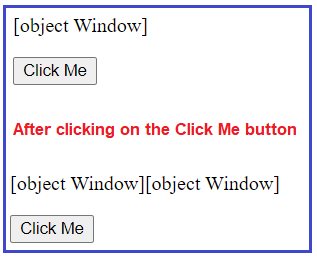Regular function refers “this” to the window scope. Whereas arrow function, do not refers this to the window, rather they execute in the scope they are created. Below is the example which elaborates it correctly.

##### Example:
```<html>
<title>JavaScript Arrow function this keyword example2</title>
<body>
<script>
window.count = 1;
//Normal function
function counter() {
this.count = 10;
setTimeout(function () {
console.log("Normal function counter : ", this.count)
}, 1000);
}
var counter = new counter();

//Arrow function
function arrowCounter() {
this.count = 10;
setTimeout(() => {
console.log("Arrow function counter : ", this.count)
}, 1000);
}
var arrowCounter = new arrowCounter();
</script>
</body>
</html>
```
###### Output: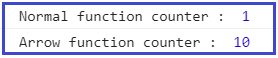Two benefits of arrow functions:

1. Shorter Syntax-the arrow function provides a convenient and short syntax to write a function expression.
2. No binding of this
1. Since Arrow functions are declared with the var keyword, they do have the characteristic of a variable. So, calling an arrow function before declaration will throw an error. That means the arrow function cannot be hoisted.
##### JavaScript Arrow function cannot be hoisted
###### Example:
```<html>
<title>JavaScript Arrow function cannot be hoisted example</title>
<body>
<script>
normalFunc();
arrowFunc();

//Normal function
function normalFunc() {
console.log("This Normal fnction can be called event before declaration");
}
//Arrow function cannot be hoisted
let arrowFunc = () => {
console.log("This Arrow function can be called only after declaration");
}
</script>
</body>
</html>
```
###### Output: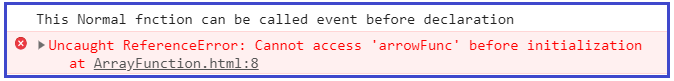##### Arrow Function Limitation

So far, we have seen arrow function with the different condition on that we can conclude that though arrow function is the alternative to the regular function, its limited and cannot be used in all situations such as

• An arrow function doesn’t have an arguments object
• The arrow function cannot be used as constructors or cannot be called using a new keyword
• An arrow function does not have its own binding to this keyword, which means use the value of this in the scope in which it was defined.